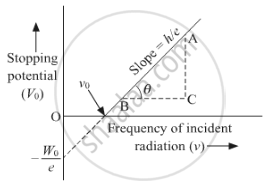# Use Einstein's photoelectric equation to show how from this graph, (i) Threshold frequency, and (ii) Planck's constant can be determined. - Physics

Use Einstein's photoelectric equation to show how from this graph,
(i) Threshold frequency, and
(ii) Planck's constant can be determined.

#### Solution

Einstein's photoelectric equation is (K. E.)max = hv - W

⇒ (K. E.)max = hv - W = eVs

⇒ eVs = hv - W

⇒ "V"_s = "h"/"e" "v" - "w"/"e""

⇒ y = mx + c

As we can see a graph can be plotted between stopping potential and frequency of incident photons.(i) Threshold frequency can be obtained from the graph where the graph cuts the x-axis, i.e. where the value of stopping potential becomes zero.

(ii) As the slope of the graph is "h"/"e", hence by calculating the slope and multiplying it by the value of charge 'e' we can also find the value of plank's constant.

Concept: Einstein’S Photoelectric Equation: Energy Quantum of Radiation
Is there an error in this question or solution?